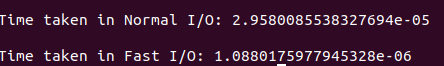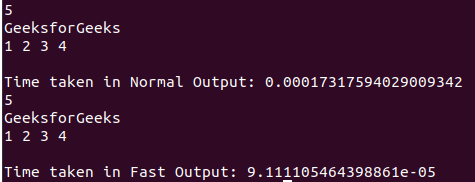# Fast I/O for Competitive Programming in Python

In Competitive Programming, it is important to read input as fast as possible to save valuable time. Input/Output in Python can be sometimes time taking in cases when the input is huge or to output any numbers of lines or a huge number of arrays(lists) line after line.

### Fast Input

Normally, the input is taken from STDIN in the form of String using input(). And this STDIN is provided in the Judge’s file. So try reading the input directly from the Judge’s file using the Operating system(os) module, and input/output (io) module. This reading can be done in the form of bytes. By using this method, integer input works normally, but for string input, it will store the string as a byte like an object. For correcting this, the string can be decoded using the decode function.

Below is the implementation for Fast I/O in Python:

## Python3

 `# Python program to illustrate the use ` `# of fast Input / Output ` `import` `io, os, time ` ` `  `# Function to take normal input ` `def` `normal_io(): ` `   `  `      ``# Stores the start time ` `    ``start ``=` `time.perf_counter() ` `     `  `    ``# Take Input ` `    ``s ``=` `input``().strip(); ` `     `  `      ``# Stores the end time ` `    ``end ``=` `time.perf_counter() ` `     `  `    ``# Print the time taken ` `    ``print``(``"\nTime taken in Normal I / O:"``, \ ` `                      ``end ``-` `start) ` ` `  `# Function for Fast Input ` `def` `fast_io(): ` `   `  `    ``# Reinitialize the Input function ` `    ``# to take input from the Byte Like  ` `    ``# objects ` `    ``input` `=` `io.BytesIO(os.read(``0``, \ ` `         ``os.fstat(``0``).st_size)).readline ` ` `  `    ``# Fast Input / Output ` `    ``start ``=` `time.perf_counter() ` ` `  `    ``# Taking input as string  ` `    ``s ``=` `input``().decode() ` `     `  `      ``# Stores the end time ` `    ``end ``=` `time.perf_counter() ` ` `  `    ``# Print the time taken ` `    ``print``(``"\nTime taken in Fast I / O:"``, \ ` `                      ``end ``-` `start) ` ` `  `# Driver Code ` `if` `__name__ ``=``=` `"__main__"``: ` ` `  `    ``# Function Call ` `    ``normal_io() ` `     `  `    ``fast_io() `

Output:### Fast Output

Instead of outputting to the STDOUT, we can try writing to the Judge’s system file. The code for that would be to use sys.stdout.write() instead of print() in Python. But remember we can only output strings using this, so convert the output to a string using str() or map().

Below is the implementation for the Fast Output:

## Python3

 `# Python program to illustrate the use ` `# of fast Input / Output ` `import` `time, sys ` ` `  `# Function to take normal input ` `def` `normal_out(): ` `   `  `      ``# Stores the start time ` `    ``start ``=` `time.perf_counter() ` ` `  `    ``# Output Integer ` `    ``n ``=` `5` `    ``print``(n) ` ` `  `    ``# Output String ` `    ``s ``=` `"GeeksforGeeks"` `    ``print``(s) ` ` `  `    ``# Output List ` `    ``arr ``=` `[``1``, ``2``, ``3``, ``4``] ` `    ``print``(``*``arr) ` ` `  `      ``# Stores the end time ` `    ``end ``=` `time.perf_counter() ` `     `  `    ``# Print the time taken ` `    ``print``(``"\nTime taken in Normal Output:"``, \ ` `                      ``end ``-` `start) ` ` `  `# Function for Fast Output ` `def` `fast_out(): ` ` `  `    ``start ``=` `time.perf_counter() ` `    ``# Output Integer ` `    ``n ``=` `5` `    ``sys.stdout.write(``str``(n)``+``"\n"``) ` ` `  `    ``# Output String ` `    ``s ``=` `"GeeksforGeeks\n"` `    ``sys.stdout.write(s) ` ` `  `    ``# Output Array ` `    ``arr ``=` `[``1``, ``2``, ``3``, ``4``] ` `    ``sys.stdout.write( ` `        ``" "``.join(``map``(``str``, arr)) ``+` `"\n"` `    ``) ` `         `  `    ``# Stores the end time ` `    ``end ``=` `time.perf_counter() ` `     `  `    ``# Print the time taken ` `    ``print``(``"\nTime taken in Fast Output:"``, \ ` `                      ``end ``-` `start) ` ` `  `# Driver Code ` `if` `__name__ ``=``=` `"__main__"``: ` ` `  `    ``# Function Call ` `    ``normal_out() ` `     `  `    ``fast_out() `

Output:My Personal Notes arrow_drop_upCheck out this Author's contributed articles.

If you like GeeksforGeeks and would like to contribute, you can also write an article using contribute.geeksforgeeks.org or mail your article to contribute@geeksforgeeks.org. See your article appearing on the GeeksforGeeks main page and help other Geeks.

Please Improve this article if you find anything incorrect by clicking on the "Improve Article" button below.